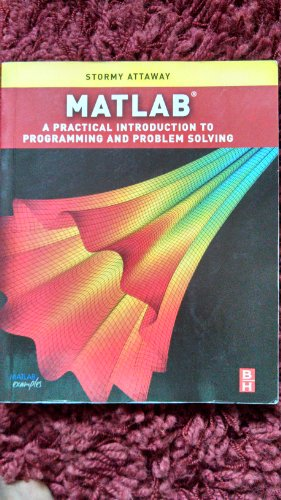# Matlab: A Practical Introduction: A Practical Introduction to Programming and Problem Solving (Hahn and Attaway Bundle)

## Attaway Ph.D. Boston University Dr., Stormy

3.87 avg rating
( 127 ratings by Goodreads )View all copies of this ISBN edition:

MATLAB: A Practical Introduction to Programming and Problem Solving discusses the basic programming concepts and skills needed for problem solving using MATLAB software. It is the only book that gives a full introduction to programming in MATLAB combined with an explanation of MATLAB’s powerful functions. The book differs from other texts in that it teaches programming concepts and the use of the built-in functions in MATLAB simultaneously. It presents programming concepts and MATLAB built-in functions side-by-side, giving students the ability to program efficiently and exploit the power of MATLAB to solve problems. The systematic, step-by-step approach, building on concepts throughout the book, facilitates easier learning.

Starting with basic programming concepts, such as variables, assignments, input/output, selection, and loop statements, problems are introduced and solved throughout the book. The book is organized into two parts. Part I covers the programming constructs and demonstrates programming versus efficient use of built-in functions to solve problems. Part II describes the applications, including plotting, image processing, and mathematics, needed in basic problem solving. The chapters feature sections called "Quick Question!" as well as practice problems designed to test knowledge about the material covered. Problems are solved using both "The Programming Concept" and "The Efficient Method," which facilitates understanding the efficient ways of using MATLAB, and also the programming concepts used in these efficient functions and operators. There are also sections on ‘common pitfalls’ and ‘programming guidelines’ that direct students towards best practice.

This book is ideal for engineers learning to program and model in MATLAB, as well as undergraduates in engineering and science taking a course on MATLAB.

* Presents programming concepts and MATLAB built-in functions side-by-side, giving students the ability to program efficiently and exploit the power of MATLAB to solve problems.
* In depth coverage of file input/output, a topic essential for many engineering applications
* Systematic, step-by-step approach, building on concepts throughout the book, facilitating easier learning
* Sections on ‘common pitfalls’ and ‘programming guidelines’ direct students towards best practice

"synopsis" may belong to another edition of this title.

Review:

The only book that gives a full introduction to programming in MATLAB combined with an explanation of MATLAB’s powerful functions, enabling engineers to fully exploit MATLAB’s power to solve engineering problems.

Synopsis:

MATLAB is now pervasive as the software tool for solving scientific and engineering problems using both its powerful built-in functions and its ability to program. Assuming no knowledge of programming, this book presents both programming concepts and MATLAB's built-in functions, providing a perfect platform for exploiting MATLAB's extensive capabilities for tackling engineering problems. It starts with programming concepts such as variables, assignments, input/output, and selection statements, moves onto loops and then solves problems using both the 'programming concept' and the 'power of MATLAB' side-by-side.In-depth coverage is given to input/output, a topic that is fundamental to many engineering applications. This title presents programming concepts and MATLAB built-in functions side-by-side, giving students the ability to program efficiently and exploit the power of MATLAB to solve problems. There is an in-depth coverage of file input/output, a topic essential for many engineering applications. Systematic, step-by-step approach, building on concepts throughout the book, facilitating easier learning.

Sections on 'common pitfalls' and 'programming guidelines' direct students towards best practice.

� 11.26

Shipping: FREE
Within U.S.A.

Destination, rates & speeds

## Matlab: A Practical Introduction to Programming and Problem Solving

ISBN 10: 0750687622 ISBN 13: 9780750687621
New Paperback Quantity: 1
Seller:
(Houston, TX, U.S.A.)

Book Description Paperback. Condition: New. Seller Inventory # 0750687622-11-21990599

� 11.26
Convert currency
Shipping: FREE
Within U.S.A.
Destination, rates & speeds

## Matlab: A Practical Introduction to Programming and Problem Solving

ISBN 10: 0750687622 ISBN 13: 9780750687621
New Softcover Quantity: 1
Seller:
GF Books, Inc.
(Hawthorne, CA, U.S.A.)

Book Description Condition: New. A+ Customer service! Satisfaction Guaranteed! Book is in NEW condition. Seller Inventory # 0750687622-2-1

� 11.27
Convert currency
Shipping: FREE
Within U.S.A.
Destination, rates & speeds

## Matlab: A Practical Introduction to Programming and Problem Solving

ISBN 10: 0750687622 ISBN 13: 9780750687621
New Softcover Quantity: 1
Seller:
My Books Store
(Tallahassee, FL, U.S.A.)

Book Description Condition: new. 1. Book is in NEW condition. Satisfaction Guaranteed! Fast Customer Service!!. Seller Inventory # MBSN0750687622

� 10.30
Convert currency
Shipping: � 3.17
Within U.S.A.
Destination, rates & speeds

## Matlab: A Practical Introduction to Programming and Problem Solving

ISBN 10: 0750687622 ISBN 13: 9780750687621
New Softcover Quantity: 1
Seller:
Irish Booksellers
(Portland, ME, U.S.A.)

Book Description Condition: New. book. Seller Inventory # M0750687622

� 29.89
Convert currency
Shipping: � 4.59
Within U.S.A.
Destination, rates & speeds

## Matlab: A Practical Introduction to Programming and Problem Solving (Hahn and Attaway Bundle) Attaway Ph.D. Boston University, Stormy

ISBN 10: 0750687622 ISBN 13: 9780750687621
New Softcover Quantity: 1
Seller:
BennettBooksLtd
(LOS ANGELES, CA, U.S.A.)

Book Description Condition: New. New. Seller Inventory # Q-0750687622